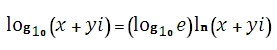# SQL Server IMLOG10 function

Updated: 27 August 2010

Use IMLOG10 to return the common (base 10) logarithm of a complex number in x + yi or x + yj text format. The base 10 logarithm of a complex number is computed as:Syntax
SELECT [wctEngineering].[wct].[IMLOG10] (
<@inumber, nvarchar(4000),>)
Arguments
@inumber
is the value at which to evaluate the function. @inumber is an expression of type varchar or of a type that can be implicitly converted to varchar and must be in the format x + yi or x + yj, where x is a real number that can be converted to float and yi (or yj) is an imaginary number, where the y part can be converted to float.
Return Types
varchar(4000)
Remarks
·         Use COMPLEX to convert real and imaginary coefficients into a complex number
Examples

select
wct.IMLOG10('7+24i')

This produces the following result
-------------------------
1.39794000867203+0.558937961295095i

(1 row(s) affected)

### SupportCopyright 2008-2023 Westclintech LLC         Privacy Policy        Terms of Service